# The data handling cycle

### Term 3 starting in week 7 :: Estimated time: 4 weeks

• Set up a statistical enquiry
• Design and criticise questionnaires
• Draw and interpret pictograms, bar charts and vertical line charts
• Draw and interpret multiple bar charts
• Draw and interpret pie charts
• Draw and interpret line graphs
• Choose the most appropriate diagram for given set of data
• Represent and interpret grouped quantitative data
• Find and interpret the range
• Compare distributions using charts

This page should remember your ticks from one visit to the next for a period of time. It does this by using Local Storage so the information is saved only on the computer you are working on right now.

## Lesson Starters

Here are some suggestions for whole-class, projectable resources which can be used at the beginnings of each lesson in this block.

### 1st Lesson#### Cars

Calculate the total cost of four cars from the information given.

### 2nd Lesson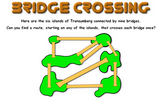#### Bridge Crossing

The classic puzzle of finding a route which crosses each bridge once.

### 3rd Lesson#### Calculator Nim

A game in which players take turns to add a single-digit number to what is already in the calculator. The winner is the player who makes the display show 30.

### 4th Lesson#### Chin-Ups

Work out the number of chin ups the characters do on the last day of the week give information about averages.

### 5th Lesson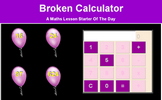#### Balloon Bursting Buttons

Use only the 1, 5 and 0 keys on a calculator to make given totals.

### 6th Lesson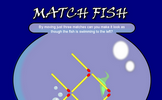#### Match Fish

A classic matchstick puzzle designed to challenge your spacial awareness.

### 7th Lesson#### Five Digits

Five digits have the same mean, median, mode and range.

### 8th Lesson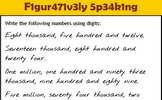#### Figuratively Speaking

Write the numbers written as words using digits.

### 9th Lesson#### Flowchart

Use the flowchart to generate a sequence of numbers. Which number will reach 1 the fastest?

### 10th Lesson#### Hours To Go

How many full hours are left till the end of the year?

### 11th Lesson#### Dancing

Work out how many people were at the dance from the clues given.

### 12th Lesson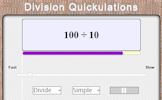#### Division Quickulations

Random division calculations appear on screen every few seconds.

Some of the Starters above are to reinforce concepts learnt, others are to introduce new ideas while others are on unrelated topics designed for retrieval practice or and opportunity to develop problem-solving skills.

White Rose ResourcesEnd of block assessments provide a quick progress check at the end of each block of learning to make sure students have understood the content covered. This Scheme of Learning was produced by White Rose Maths and is used here with permission granted on 30th June 2021.For All: